# Patch Processing

The following shows some simple examples of patch processing. See the proc module documentation for a list of processing functions.

# Basic

There are several “basic” processing functions which manipulate the patch metadata, shape, etc. Many of these are covered in the patch tutorial, but here are a few that aren’t:

## Transpose

The `transpose` patch function patch function simply transposes the dimensions of the patch, either by rotating the dimensions or to a new specified dimension.

``````import dascore as dc

patch = dc.get_example_patch()
print(f"dims before transpose: {patch.dims}")
transposed = patch.transpose()
print(f"dims after transpose: {transposed.dims}")
# dims can be manually specified as well
transposed = patch.transpose("time", "distance")``````
``````dims before transpose: ('distance', 'time')
dims after transpose: ('time', 'distance')``````

## Squeeze

The `squeeze` patch function removes dimensions which have a single value (just like numpy.squeeze).

``````import dascore as dc

patch = dc.get_example_patch()
# select first distance value to make distance dim flat.
flat_patch = patch.iselect(distance=0)
print(f"Pre-squeeze dims: {flat_patch.shape}")

squeezed = flat_patch.squeeze()
print(f"Post-squeeze dims: {squeezed.shape}")``````
``````Pre-squeeze dims: (1, 2000)
Post-squeeze dims: (2000,)``````

## Dropna

The `dropna` patch function patch function drops “nullish” values from a given label.

``````import numpy as np

import dascore as dc

patch = dc.get_example_patch()

# create a patch with nan values
data = np.array(patch.data)
data[:, 0] = np.NaN
patch_with_nan = patch.new(data=data)

# drop Nan along axis 1
dim = patch_with_nan.dims
no_na_patch = patch_with_nan.dropna(dim)

assert not np.any(np.isnan(no_na_patch.data))``````

# Decimate

The decimate patch function decimates a `Patch` along a given axis while by default performing low-pass filtering to avoid aliasing.

## Data creation

First, we create a patch composed of two sine waves; one above the new decimation frequency and one below.

``````import dascore as dc

patch = dc.examples.get_example_patch(
"sin_wav",
sample_rate=1000,
frequency=[200, 10],
channel_count=2,
)
patch.viz.wiggle(show=True);``````
``OverflowError: int too big to convert``
``<Figure size 672x480 with 1 Axes>``

## IIR filter

Next we decimate by 10x using IIR filter

``````decimated_iir = patch.decimate(time=10, filter_type='iir')
decimated_iir.viz.wiggle(show=True);``````
``OverflowError: int too big to convert``
``<Figure size 672x480 with 1 Axes>``

Notice the lowpass filter removed the 200 Hz signal and only the 10Hz wave remains.

## FIR filter

Next we decimate by 10x using FIR filter.

``````decimated_fir = patch.decimate(time=10, filter_type='fir')
decimated_fir.viz.wiggle(show=True);``````
``OverflowError: int too big to convert``
``<Figure size 672x480 with 1 Axes>``

## No Filter

Next, we decimate without a filter to purposely induce aliasing.

``````decimated_no_filt = patch.decimate(time=10, filter_type=None)
decimated_no_filt.viz.wiggle(show=True);``````
``OverflowError: int too big to convert``
``<Figure size 672x480 with 1 Axes>``

# Taper

The taper function is used to gently taper the edges of a patch dimension to zero. To see this, let’s create a patch of all 1s and apply the taper function

``````import numpy as np
import dascore as dc

_patch = dc.get_example_patch()
patch_ones = _patch.new(data=np.ones_like(_patch.data))``````

The following code will apply a 10% taper, meaning the first and last 10% of the samples, along the time dimension.

``patch_ones.taper(time=0.1).viz.waterfall();``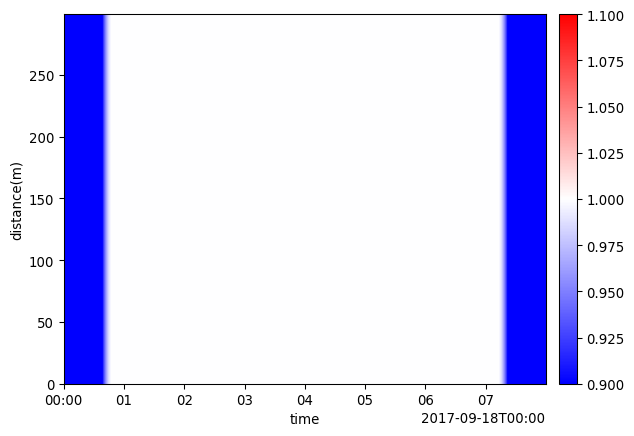Of course, any dimensions works, and passing a tuple of values enables different amounts of tapering for each end of the dimension.

``patch_ones.taper(distance=(0.1, 0.3)).viz.waterfall();``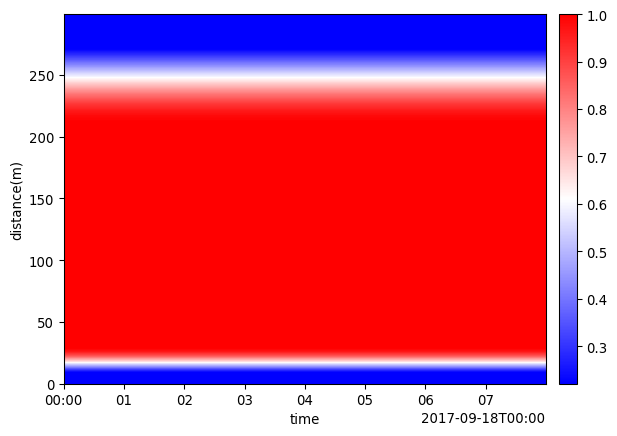Either end can also be `None` to indicated tapering on a single side of the patch dimension.

``patch_ones.taper(distance=(None, 0.1)).viz.waterfall();``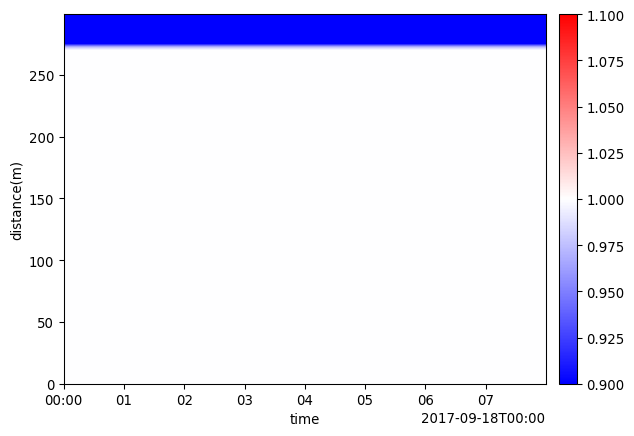## Managing Edge Effects

One of the main applications of tapering is to mitigate edge artefacts, or “edge effects” associated with several other types of processing, including filtering. Consider the following:

### Bandpass filtering without tapering

``````import dascore as dc

patch = (
dc.get_example_patch('example_event_1')
.pass_filter(time=(None, 300))
)

patch.viz.waterfall(show=True, scale=0.04);``````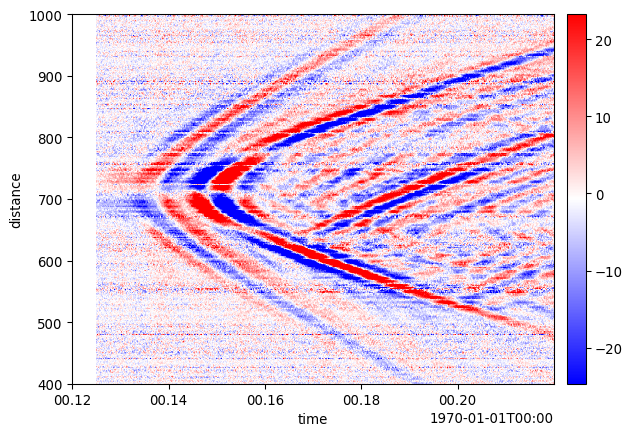### Bandpass filtering with tapering

``````import dascore as dc

patch = (
dc.get_example_patch('example_event_1')
.taper(time=0.05)
.pass_filter(time=(None, 300))
)

patch.viz.waterfall(show=True, scale=0.15);``````# Rolling

The rolling patch function implements moving window operators similar to pandas rolling. It is useful for smoothing, calculating aggregated statistics, etc.

Here is an example of using a rolling mean to smooth along the time axis for an example event:

``````import dascore as dc

patch = dc.get_example_patch("example_event_1")
# apply moving mean window over every 10 samples
dt = patch.get_coord('time').step

smoothed = patch.rolling(time=50*dt).mean()
smoothed.viz.waterfall(scale=.1);``````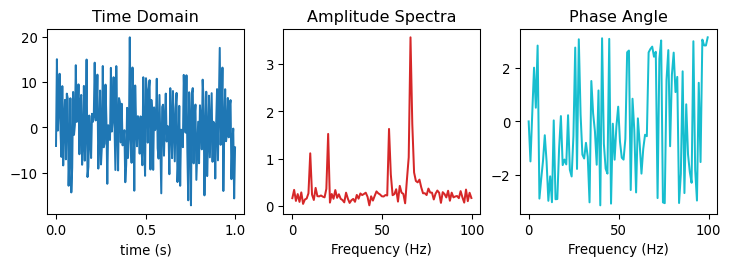Notice the NaN values at the start of the time axis. These can be trimmed with `Patch`.dropna.